# Reasoning MCQ - 28

## 30 Questions MCQ Test Reasoning Aptitude for Competitive Examinations | Reasoning MCQ - 28

Description
Attempt Reasoning MCQ - 28 | 30 questions in 30 minutes | Mock test for LR preparation | Free important questions MCQ to study Reasoning Aptitude for Competitive Examinations for LR Exam | Download free PDF with solutions
QUESTION: 1

### Directions : Each of the questions below consists of a question and two statements numbered I and II given below it. You have to decide whether the data provided in the statements are sufficient to answer the question.Read both the statement and give answer: Q. P, Q, R, S and T are sitting around a circle facing towards its centre. Who is second to the right of P? I. R is to the immediate left of T and second to the right of S. II. Q is to the immediate right of S and third to the left of P.

Solution: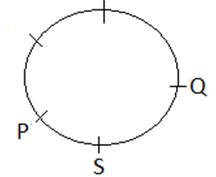QUESTION: 2

### Directions : Each of the questions below consists of a question and two statements numbered I and II given below it. You have to decide whether the data provided in the statements are sufficient to answer the question.Read both the statement and give answer: Q. Who is sitting to the immediate right to Manisha among five friends sitting around a circle facing the centre? I. Sherya is sitting exactly between Kajal and Sneha and Raj is sitting to the immediate right of Sneha. II. Manisha is sitting exactly between Kajal and Raj and Sherya is sitting to the immediate right of Kajal.

Solution: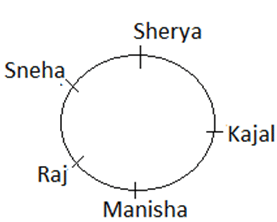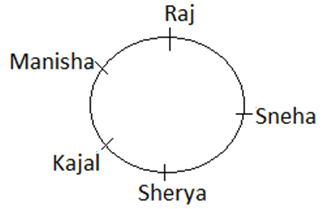QUESTION: 3

### Directions : Each of the questions below consists of a question and two statements numbered I and II given below it. You have to decide whether the data provided in the statements are sufficient to answer the question.Read both the statement and give answer: Q. Who is to the immediate right of P among five persons P, Q, R, S and T facing North? I. R is third to the left of Q and P is second to the right of R. II. Q is to the immediate left of T who is second to the right of P.

Solution: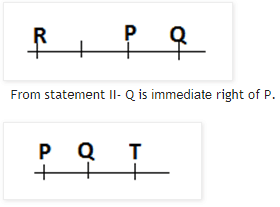QUESTION: 4

Directions : Each of the questions below consists of a question and two statements numbered I and II given below it. You have to decide whether the data provided in the statements are sufficient to answer the question.Read both the statement and give answer:

Q.

What is Manoj’s rank from the top in a class of 50 students?

I. Ankur is 6 ranks below Manoj and is twenty-ninth from the bottom.

II. Akhil is 4 ranks above Manoj and is thirty-fifth from the bottom.

Solution: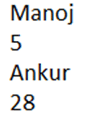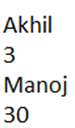QUESTION: 5

Directions : Each of the questions below consists of a question and two statements numbered I and II given below it. You have to decide whether the data provided in the statements are sufficient to answer the question.Read both the statement and give answer:

Q.

Point X is in which direction with respect to Y?

I. Point Z is at equal distance from both point X and point Y.

II. Starting from point X, a man starts walking towards east and after walking 5 km, he takes a right turn and walks 5 km again to reach a point A. He then takes a right turn again and walks 5 km to reach point Y.

Solution: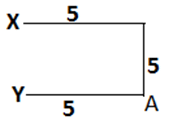QUESTION: 6

Directions : Each of the questions below consists of a question and three statements numbered I, II and III given below it. You have to decide whether the data provided in the statements are sufficient to answer the question. Read all the three statements and Give answer.

Q.

Eight students A, B, C, D, M, N, O and P are sitting around a circle facing to the centre of the table. Who sits fourth to right of C?

I. C is seated third to the right of A and is not near to M. N is seated second to the right B.

II. A is seated second to the right of P, who is seated third to the right of D. O is seated immediate left of N.

III. D is seated second to the left of M and is third to right of O. C is an immediate neighbor of P.

Solution:
QUESTION: 7

Directions : Each of the questions below consists of a question and three statements numbered I, II and III given below it. You have to decide whether the data provided in the statements are sufficient to answer the question. Read all the three statements and Give answer.

Q.

How many sons does Pallavi have?

(I) Ankita’s father Darshan is husband of Pallavi.

(II) Out of the three children whom Darshan has only one girl.

(III) Viral and Sparsh is brother of Ankita.

Solution: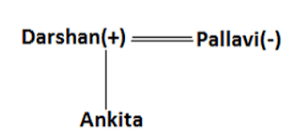QUESTION: 8

Directions : Each of the questions below consists of a question and three statements numbered I, II and III given below it. You have to decide whether the data provided in the statements are sufficient to answer the question. Read all the three statements and Give answer

Q.

Point D is in which direction with respect to Point B?

I. Point A is to the west of Point B. Point C is to the north of Point B. Point D is to the south of Point A.

II. Point G is to the south of Point D and west of Point B.

III. Point A is to the west of Point B. Point B is exactly midway between Point A and E. Point F is to the south of Point E. Point D is to the east of Point F.

Solution: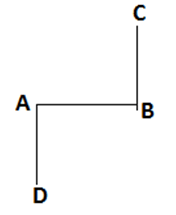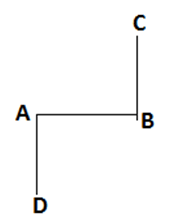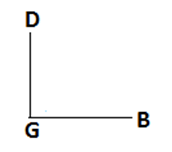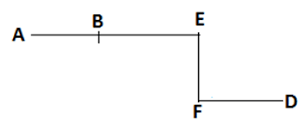QUESTION: 9

Directions : Each of the questions below consists of a question and three statements numbered I, II and III given below it. You have to decide whether the data provided in the statements are sufficient to answer the question. Read all the three statements and Give answer.

Q.

How is ‘one’ coded in a coded language?

I. ‘one of its kind, is coded as ‘zo pi ko fe’ and ‘in kind and cash’ is coded as ‘ga to ru ko’.

II. ‘its point for origin’ is coded as ‘ba le fe mi’ and ‘make a point clear’ is coded as ‘yu si mi de’.

III. ‘make money and cash’ is coded as ‘to mi ru hy’ and ‘money of various kind’ is coded as ‘qu ko zo hy’

Solution:
QUESTION: 10

Directions : Each of the questions below consists of a question and three statements numbered I, II and III given below it. You have to decide whether the data provided in the statements are sufficient to answer the question. Read all the three statements and Give answer

Q.

Are all the four friends, viz A, B, C and D, who are sitting around a circular table, facing the centre?

I. B sits second to the right of D. D faces the centre. C sits on the immediate right of both B and D.

II. A sits on the immediate left of B. C is not an immediate neighbour of A. C sits on the immediate right of D.

III. D is an immediate neighbour of both A and C. B sits on the immediate left of A. C sits on the immediate right of

Solution: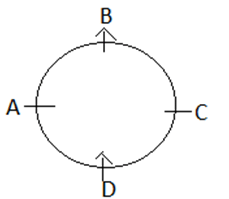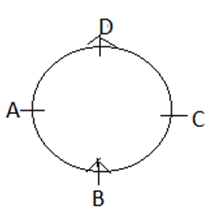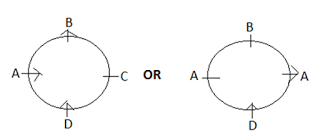QUESTION: 11

Directions : Each of the following questions consists of six statements followed by options consisting of three statements put together in a specific order. Choose the options that indicates a combination where the third statement can be logically deduced from the first two statements and that option will be your answer.

Q1.

i. All man is boy.

ii. Some boy is toy.

iii. All toy is game.

iv. Some game is play.

v. Some man is play.

vi. Some toy is man

Solution:
QUESTION: 12

Directions : Each of the following questions consists of six statements followed by options consisting of three statements put together in a specific order. Choose the options that indicates a combination where the third statement can be logically deduced from the first two statements and that option will be your answer.

Q.

i. All black is white.

ii. Some black is pink.

iii. Some pink is black.

iv.All black is yellow.

v. Some yellow is white.

vi. Some white is pink.

Solution: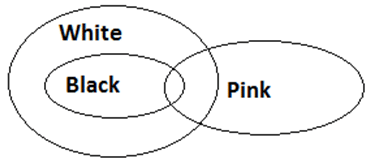QUESTION: 13

Directions : Each of the following questions consists of six statements followed by options consisting of three statements put together in a specific order. Choose the options that indicates a combination where the third statement can be logically deduced from the first two statements and that option will be your answer.

Q.

i.No train is car.

ii.Some bus is train.

iii.No train is green.

iv. All train is bus.

v. Some green is tree.

vi. No bus is car.

Solution: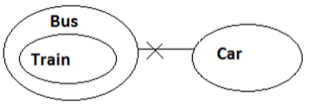QUESTION: 14

Directions : In each question, a set of six statements is given, followed by five answer choices. Each of the answer choices has a combination of three statements from the given set of six statements. You are required to identify the answer choice in which the statements are logically related.

Q.

Statements:

I.Some school is bus.

II. No school is train.

III. Some train is laptop.

IV. No laptop is copy.

V. All train is car.

VI. No bus is train.

Solution: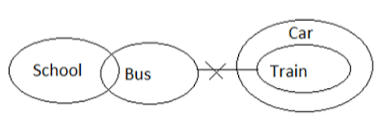QUESTION: 15

Directions : In each question, a set of six statements is given, followed by five answer choices. Each of the answer choices has a combination of three statements from the given set of six statements. You are required to identify the answer choice in which the statements are logically related.

Q.

Statements:

I. Some man is boy.

II. No boy is toy.

III. Some toy is red.

IV. All red is boy.

V. No boy is black.

VI. All car is toy.

Solution: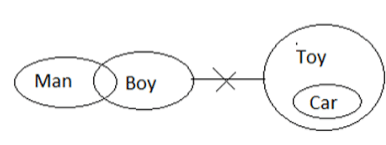QUESTION: 16

Directions : In each question, a set of six statements is given, followed by five answer choices. Each of the answer choices has a combination of three statements from the given set of six statements. You are required to identify the answer choice in which the statements are logically related.

Q.

Statements:

I. No cat is deer.

II. Some mouse is rat.

III. All deer is cow.

IV. Some dog is deer

V. All cat is rat.

VI. Some dog is cat.

Solution: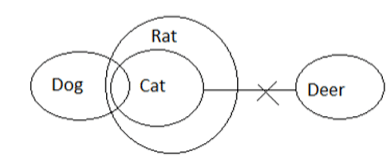QUESTION: 17

Directions :

In the questions below are given two conclusions followed by four set of statements. You have to choose the correct set of statements that logically satisfies given conclusions either definitely or possibly. Assume the given statements to be true even if they seem to be at variance from commonly known facts.

Q.

Conclusions: Some Tyre is bus. Some truck is not tyre.

Statements:

Solution: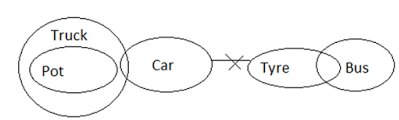QUESTION: 18

Directions :

In the questions below are given two conclusions followed by four set of statements. You have to choose the correct set of statements that logically satisfies given conclusions either definitely or possibly. Assume the given statements to be true even if they seem to be at variance from commonly known facts.

Q.

Conclusions: Some finger is hair. Some leg is not hair.

Statements:

Solution: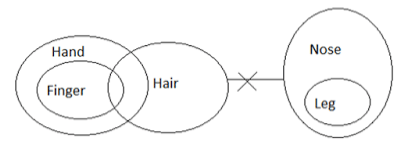QUESTION: 19

Directions :

In the questions below are given two conclusions followed by four set of statements. You have to choose the correct set of statements that logically satisfies given conclusions either definitely or possibly. Assume the given statements to be true even if they seem to be at variance from commonly known facts.

Q.

Conclusions: Some harvest are not green. No Forest is green.

Statements:

Solution: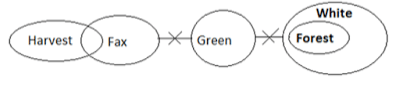QUESTION: 20

Directions : Question consists of five statements followed by five conclusions. Consider the given statements to be true even if they seem to be at variance with commonly known facts. Read all the conclusions and then decide which of the given conclusions does not logically follow from the given statements using all statements together.

Q.

Statements: Some lotto is rupani. All rupani is sparx. No sparx is woodland. Some woodland is redtape. All redtape is leecooper.

Conclusions:

Solution: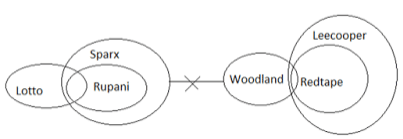QUESTION: 21

Directions : Question consists of five statements followed by five conclusions. Consider the given statements to be true even if they seem to be at variance with commonly known facts. Read all the conclusions and then decide which of the given conclusions does not logically follow from the given statements using all statements together.

Q.

Statements: All jeans is shirt. All shirt is pant. Some shirt is cloth. All cloth is suit. Some cloth is saree.

Conclusions:

Solution: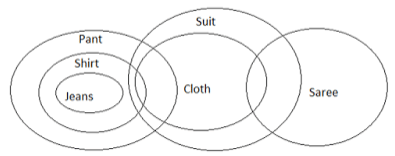QUESTION: 22

Directions : Question consists of five statements followed by five conclusions. Consider the given statements to be true even if they seem to be at variance with commonly known facts. Read all the conclusions and then decide which of the given conclusions does not logically follow from the given statements using all statements together.

Q.

Statements: No line is paper. All paper is point. No point is center. Some center is main. All main is gate.

Conclusions:

Solution: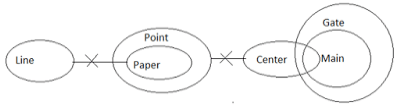QUESTION: 23

Directions : In each of the questions below are given four statements followed by four conclusions numbered I, II, III and IV. You have to assume everything in the statements to be true even if they seem to be at variance with commonly known facts and then decide which of the four given conclusions logically follows from the statements, disregarding commonly known facts, and select the appropriate answer.

Q.

Statement:

No green is people.

All people are animals.

All animals are plants.

All plants are roots.

Conclusions:

I. No green is a plant.

II. All people are plants.

III. Some green are plants.

IV. All plants are green.

Solution: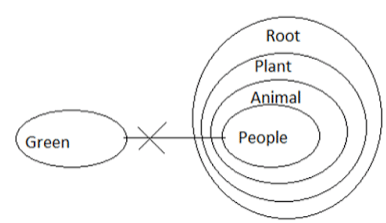QUESTION: 24

Directions : In each of the questions below are given four statements followed by four conclusions numbered I, II, III and IV. You have to assume everything in the statements to be true even if they seem to be at variance with commonly known facts and then decide which of the four given conclusions logically follows from the statements, disregarding commonly known facts, and select the appropriate answer.

Q.

Statement:

Some jobs are result.

All jobs are works.

Conclusions:

I. At least some works are jobs.

II. No result is a trade.

III. No profession is a work.

IV. At least some works are professions.

Solution: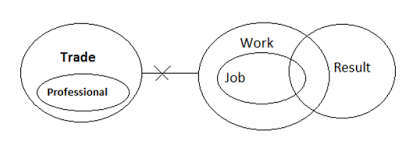QUESTION: 25

Directions : In each of the questions below are given four statements followed by four conclusions numbered I, II, III and IV. You have to assume everything in the statements to be true even if they seem to be at variance with commonly known facts and then decide which of the four given conclusions logically follows from the statements, disregarding commonly known facts, and select the appropriate answer.

Q.

Statement:

All man are goat.

No goat is a score.

All letters are scores.

All scores are characters.

Conclusions:

I. At least some letters are goat.

II. At least some scores are goat.

III. At least some characters are man.

IV. Some characters are letters.

Solution:QUESTION: 26

Directions : Study the following information to answer the given questions:

In a certain code,

“the news glaring holes”  is written as ‘ 4E@ 13I#  22L#  19T#’

“for consular access and” is written as ‘13A#  12F#   26L@  8E@’

“splice tutor mark” is written as ‘  9A@  24I@ 12T#’

Q.

What must be the code for “PURSE”?

Solution: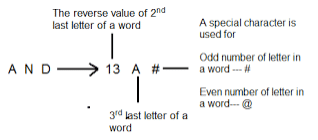QUESTION: 27

Directions : Study the following information to answer the given questions:

In a certain code,

“the news glaring holes”  is written as ‘ 4E@ 13I#  22L#  19T#’

“for consular access and” is written as ‘13A#  12F#   26L@  8E@’

“splice tutor mark” is written as ‘  9A@  24I@ 12T#’

Q.

What is the code of “power purchase”?

Solution:QUESTION: 28

Directions : Study the following information to answer the given questions:

In a certain code,

“the news glaring holes”  is written as ‘ 4E@ 13I#  22L#  19T#’

“for consular access and” is written as ‘13A#  12F#   26L@  8E@’

“splice tutor mark” is written as ‘  9A@  24I@ 12T#’

Q.

If all the vowels of the word “APPLE” are removed, then what would be the code for the resulting word?

Solution:QUESTION: 29

Directions : Study the following information to answer the given questions:

In a certain code,

“the news glaring holes”  is written as ‘ 4E@ 13I#  22L#  19T#’

“for consular access and” is written as ‘13A#  12F#   26L@  8E@’

“splice tutor mark” is written as ‘  9A@  24I@ 12T#’

Q.

Which of the following can be coded as “9A#”?

Solution:QUESTION: 30

​ Directions : Study the following information to answer the given questions:

In a certain code,

“the news glaring holes”  is written as ‘ 4E@ 13I#  22L#  19T#’

“for consular access and” is written as ‘13A#  12F#   26L@  8E@’

“splice tutor mark” is written as ‘  9A@  24I@ 12T#’

Q.

What will be the code for “DARKED”?

Solution:Use Code STAYHOME200 and get INR 200 additional OFF Use Coupon Code